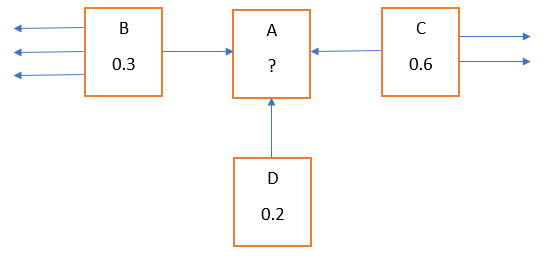What is Google's page rank algorithm? How to implement page rank algorithm? How search engine sorts results?

 Category: Machine Learning Tags:Page rank algorithm in python

Introduction

In our previous article, we have seen how to create crawler and a simple search, in this article I will show you how to implement page rank algorithm to get better search results. Page rank algorithm was developed by google developers and used by other search engines too. I recommend you first to go through previous article.

Page Rank Algorithm: Rank of a page is calculated by calculating importance of a page and a page is as much important as many other page link to it. We will have to generate a score for every page by dividing it’s rank by total links on the page, for example rank of C is 0.6 and total links on page are 3 so score will be 0.6/3 = 0.2. We consider two thigs here one is minimum score which is 0.15, the second one is dumping factor which is 0.85. Dumping factor 0.85 means 85% of probability a user will click on any link available on page (rest 15% probability he might not click on any link).Fig 1: Page rank algorithm

So, A’s rank

= 0.15 + 0.85*(B’s PR/Total links on B + C’s PR/Total links on C + D’s PR/Total links on D)

= 0.15 + 0.85*(0.3/4 + 0.6/3 + 0.2/1)

= 0.15 + 0.85*0.475

= 0.5537

Implementation

We have created SearchEngine database in previous article and here we will be adding one more table called PageRank in this database. PageRank table will store calculated rank of every URL, below is the script to create table:

CREATE TABLE PageRank ( UrlId INTEGER PRIMARY KEY, Rank INTEGER, FOREIGN KEY(UrlId) REFERENCES Url(Id) )

Here we will have a problem, initially we will not be having rank for any page and until we have rank of page B, C and D we will not be able to calculate rank of A. To resolve this, we will set rank of all pages to 1 and iterate algorithm for n number of times, suppose for 10 links 20 iteration will be sufficient.

Now let’s write code for page rank algorithm, we had created a class searchEngine in previous article, we will add a method calculatePageRank in same class:

def calculatePageRank(self, iterations=20):
access = dataAccess(self.database)
#Resetting rank to 1
access.executeCommand('DELETE FROM PageRank', None)
access.executeCommand('INSERT INTO PageRank(UrlId, Rank) SELECT Id, 1 FROM Url', None)
for i in range(iterations):
print('Iteration ',i)
urlIds = access.selectCommand('SELECT Id FROM Url')
for urlId in urlIds:
#Minimum rank
pageRank = 0.15
#Which pages link to current page
fromUrlIds = access.selectCommand('SELECT DISTINCT FromId FROM Link WHERE ToId=%d'%urlId)
for fromUrlId in fromUrlIds:
#Rank of page
rank = access.selectCommand('SELECT Rank FROM PageRank WHERE UrlId=%d'%fromUrlId)
#Total links on page
numberOfLinks = access.selectCommand('SELECT COUNT(*) FROM Link WHERE FromId=%d'%fromUrlId)
#Calculation
pageRank += 0.85 * rank/numberOfLinks
#Storing rank
access.executeCommand('UPDATE PageRank SET Rank={0} WHERE UrlId={1}'.format(pageRank,urlId), None)

Above method will calculate page rank and store it in database now we will create a method to retrieve page rank of each page and sort it based on rank.

def sortResultsByPageRank(self, rows):
access = dataAccess(self.database)
#Distinct results
resultDict = dict([(row, 0) for row in rows])
for result in resultDict.items():
#getting rank of each page
pageRank = access.selectCommand('SELECT Rank from Url u INNER JOIN PageRank pr on u.Id = pr.UrlId WHERE u.Link like \'%s\''%result)
resultDict[result] = pageRank
#Sorting based on rank
sortedResult = sorted(resultDict.items(), key=operator.itemgetter(1), reverse=True)
return sortedResult

To get desired results we first have to crawl a website like we already did in last article, I’m not demonstrating that here then we have to run calculatePageRank method so it will calculate rank of each page and store it in database:

searchEng = searchEngine('D:\\SearchEngine.db')
searchEng.calculatePageRank()

Now let’s search for a keyword “tea” and get results based on page rank:

searchEng = searchEngine('D:\\SearchEngine.db')
results = searchEng.search('tea')
sortedResultsByPageRank = searchEng.sortResultsByPageRank(results)
print(sortedResultsByPageRank)

Output:

[

('https://www.practiceselenium.com/welcome.html', 0.26724822044559043),

('https://www.practiceselenium.com/our-passion.html', 0.2672482204455904),

('https://www.practiceselenium.com/let-s-talk-tea.html', 0.2672482204224549),

('https://www.practiceselenium.com/check-out.html', 0.2672482204039138),

('https://www.practiceselenium.com', 0.15)

]

Conclusion

Page ranking algorithm can be used to sort the search results, time to time this algorithm is evolved with many other factors any many other search engine used it. There are many other algorithms used by google to give relevant results and some are kept secret.

 Like0 PeopleNikhil Joshi Ceo & Founder at Dotnetlovers Atricles: 133 Questions: 9 Given Best Solutions: 9 *

Reference:

programming collective intelligence - by Toby Segaran and published by O'Reilly

Login via:x